# Selina Solutions Concise Maths Class 7 Chapter 9: Profit, Loss and Discount Exercise 9C

Selina Solutions Concise Maths Class 7 Chapter 9 Profit, Loss and Discount Exercise 9C explains students about the concept of discount and method of determining them. The reduction in the marked price of an object is called a discount. Students can refer to the solutions, which are available for online and offline studying purposes and get their doubts cleared on the spot. It improves time management skills among students, which are important from the exam point of view. Students can use Selina Solutions Concise Maths Class 7 Chapter 9 Profit, Loss and Discount Exercise 9C PDF, from the links which are available below.

## Selina Solutions Concise Maths Class 7 Chapter 9: Profit, Loss and Discount Exercise 9C Download PDF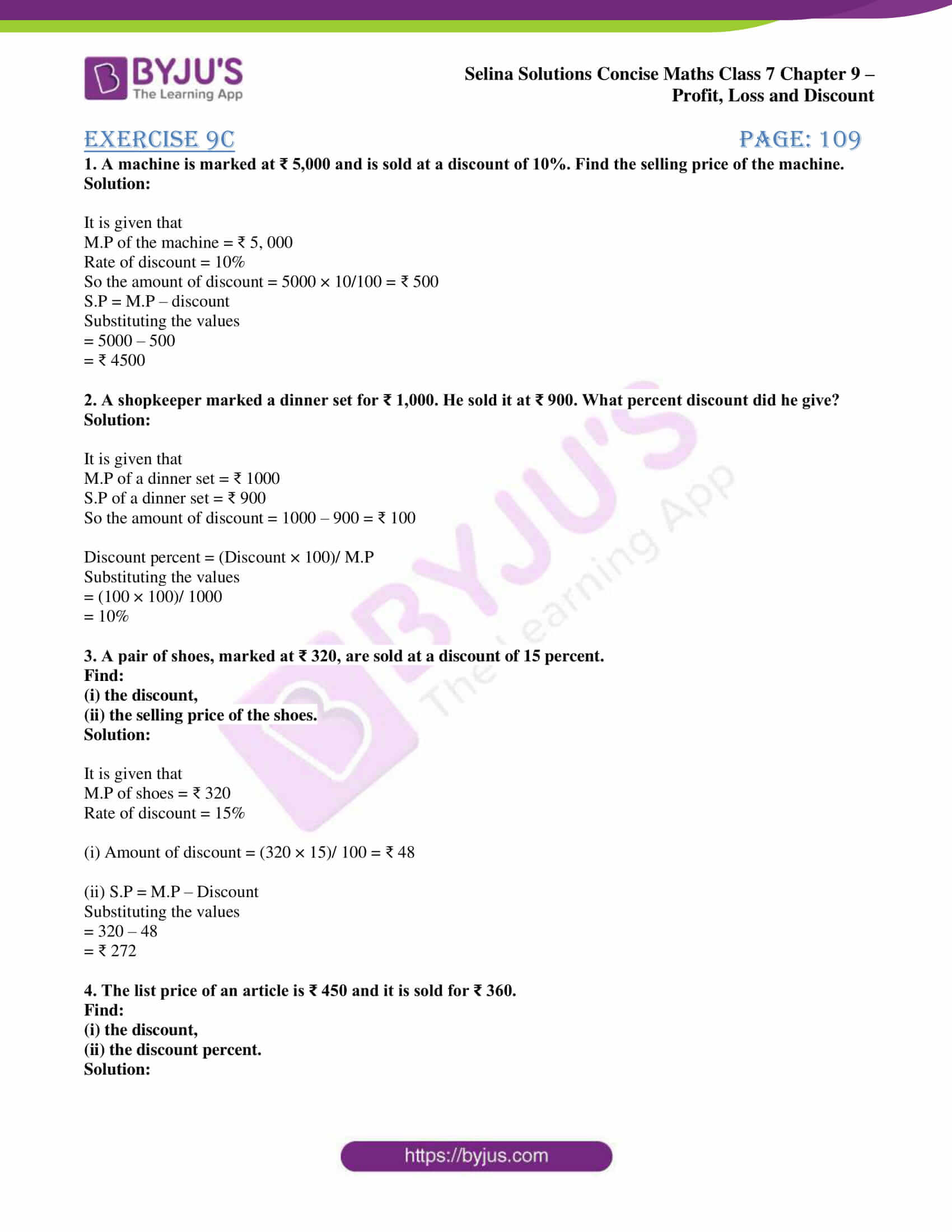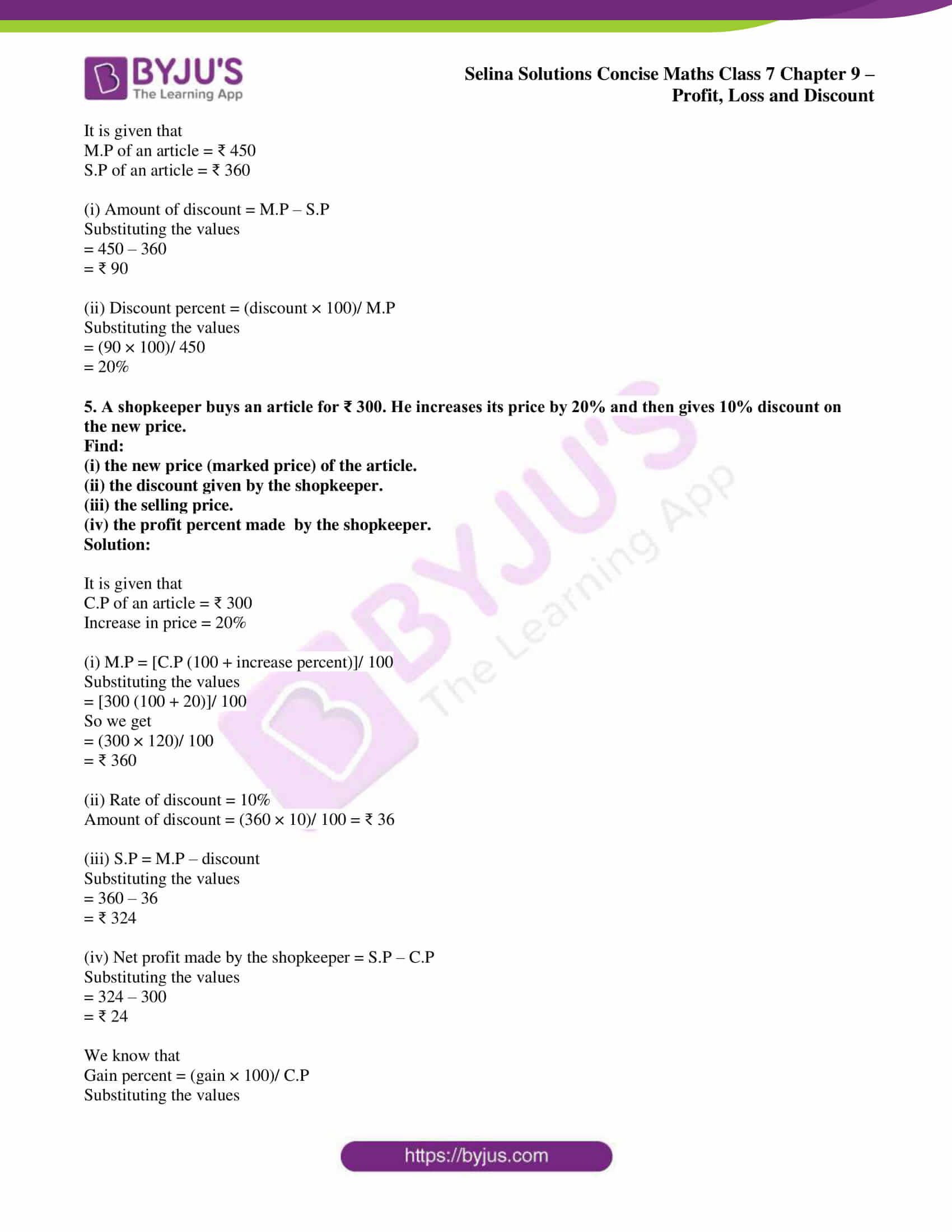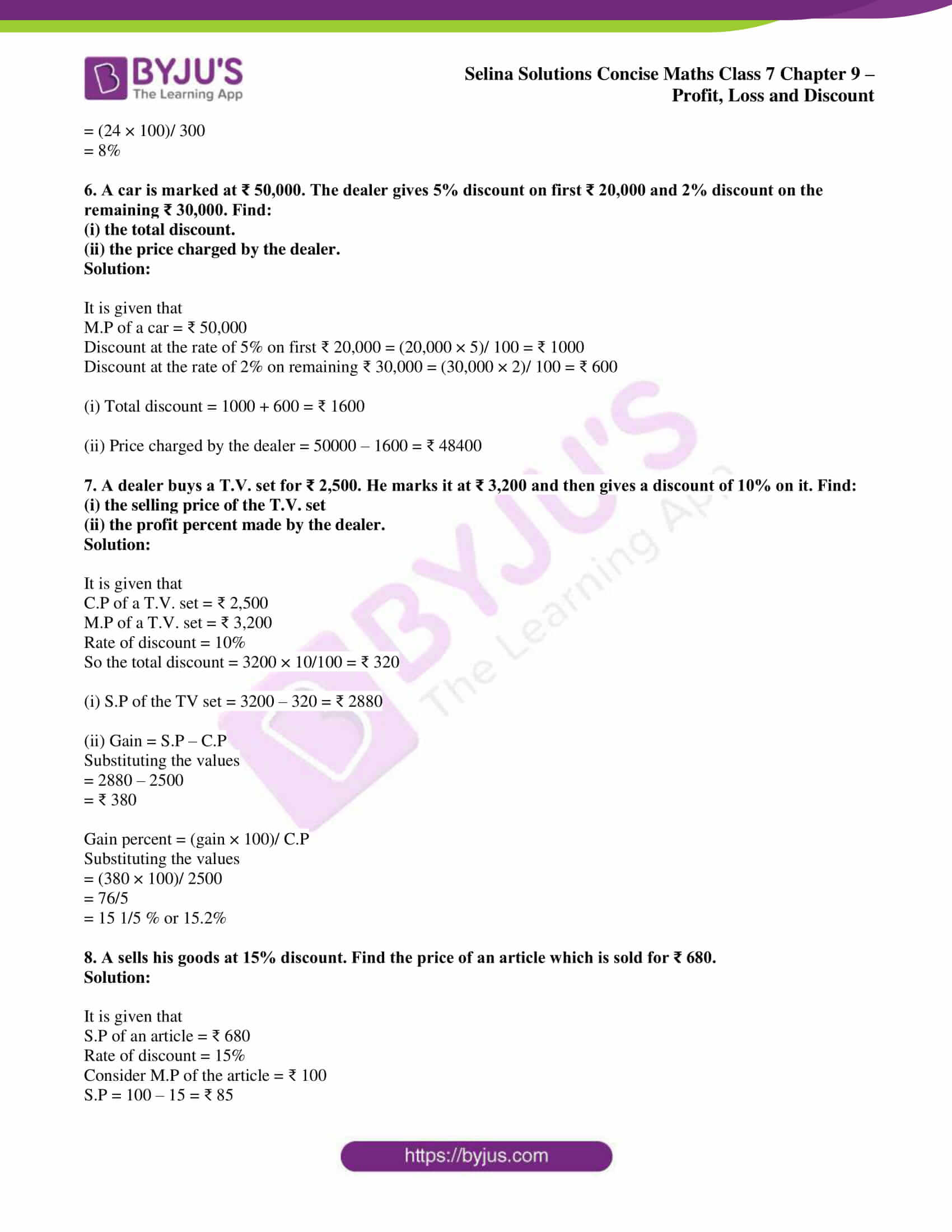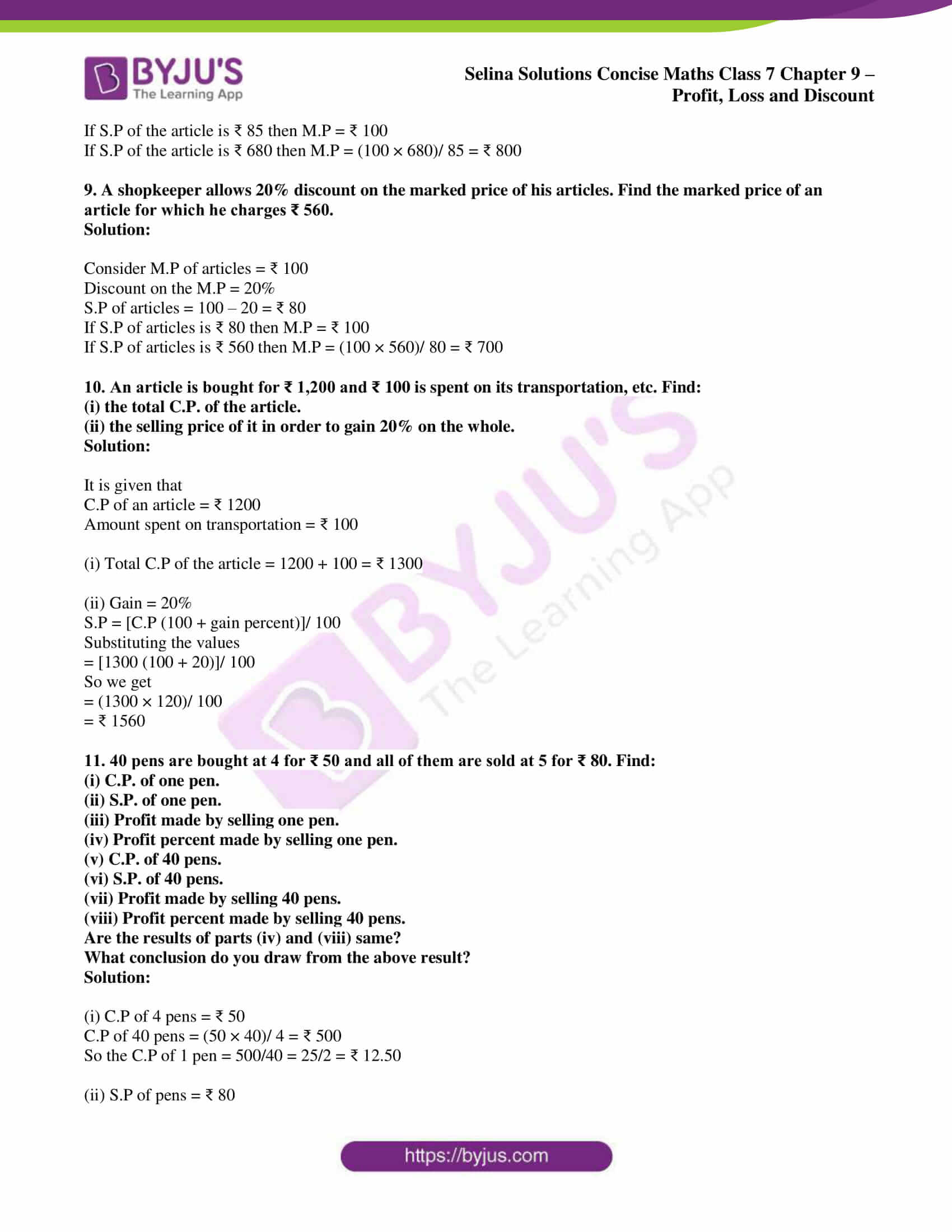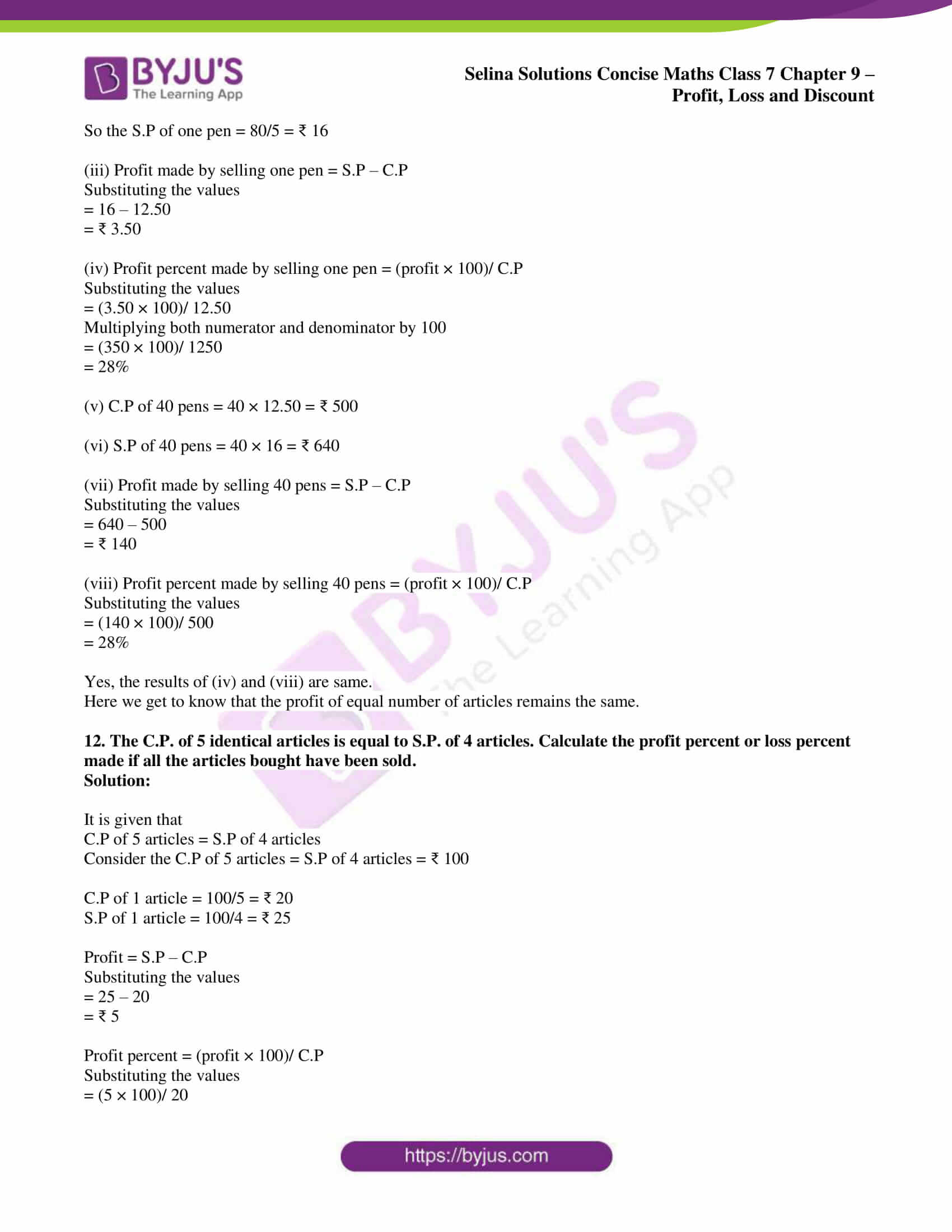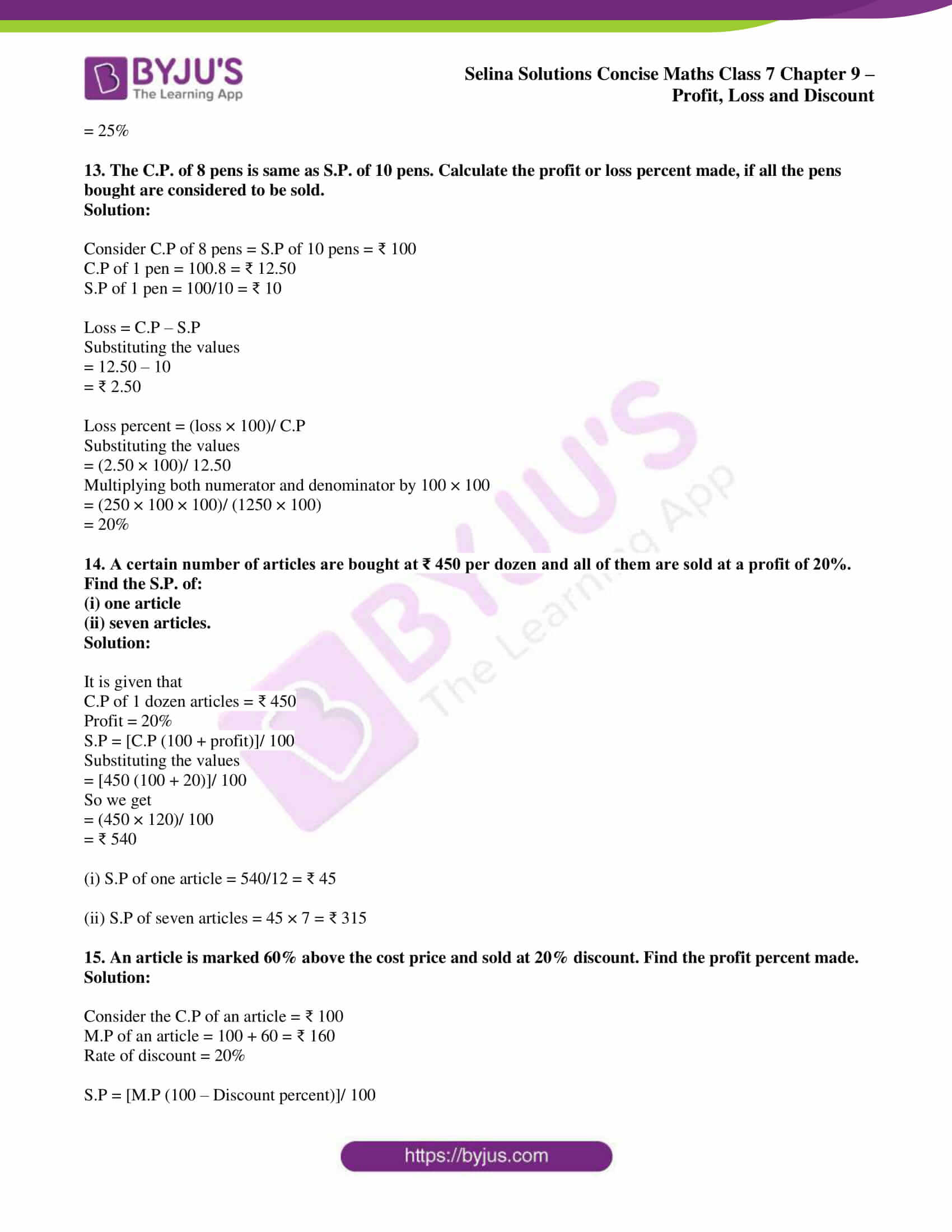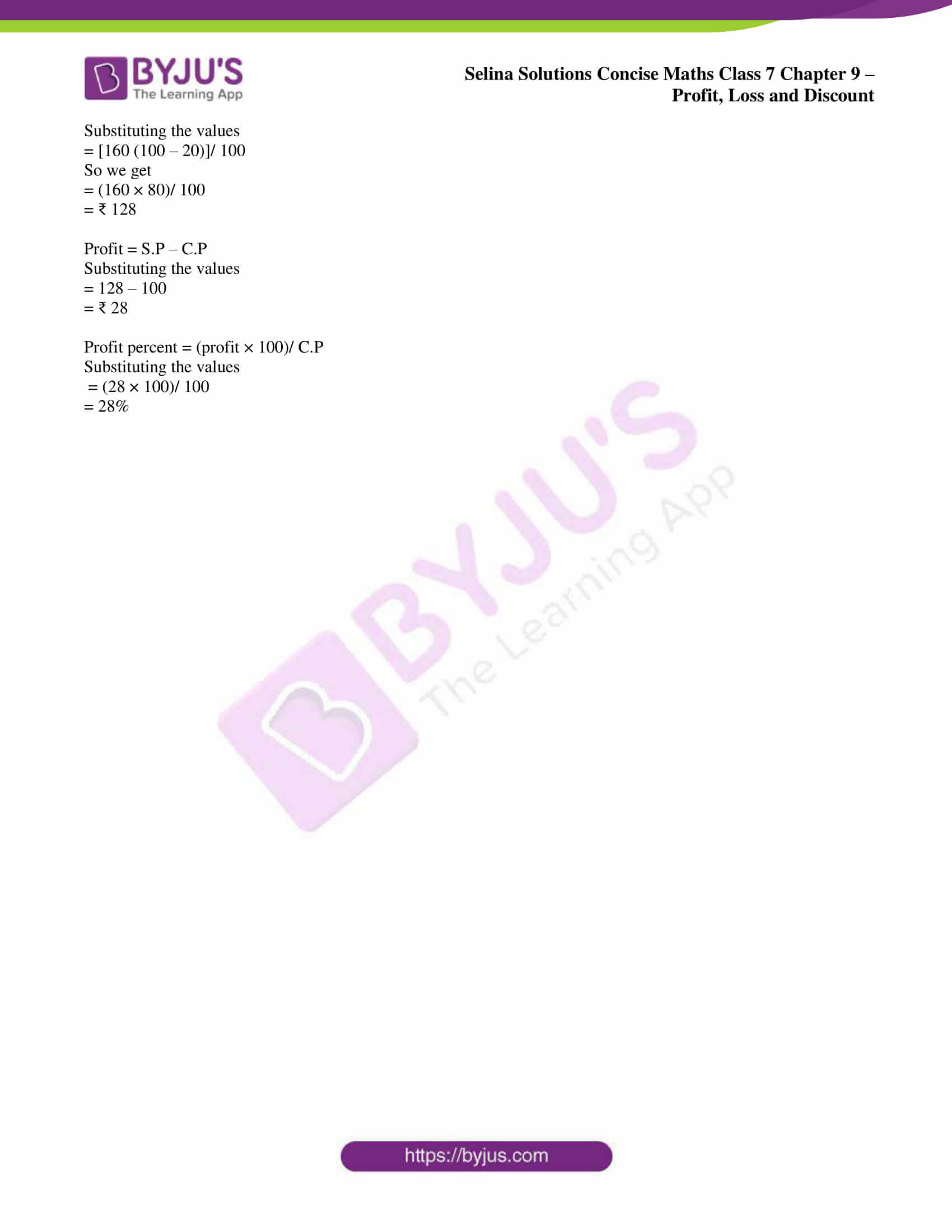### Access Selina Solutions Concise Maths Class 7 Chapter 9: Profit, Loss and Discount Exercise 9C

#### Exercise 9C page: 109

1. A machine is marked at ₹ 5,000 and is sold at a discount of 10%. Find the selling price of the machine.

Solution:

It is given that

M.P of the machine = ₹ 5, 000

Rate of discount = 10%

So the amount of discount = 5000 × 10/100 = ₹ 500

S.P = M.P – discount

Substituting the values

= 5000 – 500

= ₹ 4500

2. A shopkeeper marked a dinner set for ₹ 1,000. He sold it at ₹ 900. What percent discount did he give?

Solution:

It is given that

M.P of a dinner set = ₹ 1000

S.P of a dinner set = ₹ 900

So the amount of discount = 1000 – 900 = ₹ 100

Discount percent = (Discount × 100)/ M.P

Substituting the values

= (100 × 100)/ 1000

= 10%

3. A pair of shoes, marked at ₹ 320, are sold at a discount of 15 percent.

Find:

(i) the discount,

(ii) the selling price of the shoes.

Solution:

It is given that

M.P of shoes = ₹ 320

Rate of discount = 15%

(i) Amount of discount = (320 × 15)/ 100 = ₹ 48

(ii) S.P = M.P – Discount

Substituting the values

= 320 – 48

= ₹ 272

4. The list price of an article is ₹ 450 and it is sold for ₹ 360.

Find:

(i) the discount,

(ii) the discount percent.

Solution:

It is given that

M.P of an article = ₹ 450

S.P of an article = ₹ 360

(i) Amount of discount = M.P – S.P

Substituting the values

= 450 – 360

= ₹ 90

(ii) Discount percent = (discount × 100)/ M.P

Substituting the values

= (90 × 100)/ 450

= 20%

5. A shopkeeper buys an article for ₹ 300. He increases its price by 20% and then gives 10% discount on the new price.

Find:

(i) the new price (marked price) of the article.

(ii) the discount given by the shopkeeper.

(iii) the selling price.

(iv) the profit percent made by the shopkeeper.

Solution:

It is given that

C.P of an article = ₹ 300

Increase in price = 20%

(i) M.P = [C.P (100 + increase percent)]/ 100

Substituting the values

= [300 (100 + 20)]/ 100

So we get

= (300 × 120)/ 100

= ₹ 360

(ii) Rate of discount = 10%

Amount of discount = (360 × 10)/ 100 = ₹ 36

(iii) S.P = M.P – discount

Substituting the values

= 360 – 36

= ₹ 324

(iv) Net profit made by the shopkeeper = S.P – C.P

Substituting the values

= 324 – 300

= ₹ 24

We know that

Gain percent = (gain × 100)/ C.P

Substituting the values

= (24 × 100)/ 300

= 8%

6. A car is marked at ₹ 50,000. The dealer gives 5% discount on first ₹ 20,000 and 2% discount on the remaining ₹ 30,000. Find:

(i) the total discount.

(ii) the price charged by the dealer.

Solution:

It is given that

M.P of a car = ₹ 50,000

Discount at the rate of 5% on first ₹ 20,000 = (20,000 × 5)/ 100 = ₹ 1000

Discount at the rate of 2% on remaining ₹ 30,000 = (30,000 × 2)/ 100 = ₹ 600

(i) Total discount = 1000 + 600 = ₹ 1600

(ii) Price charged by the dealer = 50000 – 1600 = ₹ 48400

7. A dealer buys a T.V. set for ₹ 2,500. He marks it at ₹ 3,200 and then gives a discount of 10% on it. Find:

(i) the selling price of the T.V. set

(ii) the profit percent made by the dealer.

Solution:

It is given that

C.P of a T.V. set = ₹ 2,500

M.P of a T.V. set = ₹ 3,200

Rate of discount = 10%

So the total discount = 3200 × 10/100 = ₹ 320

(i) S.P of the TV set = 3200 – 320 = ₹ 2880

(ii) Gain = S.P – C.P

Substituting the values

= 2880 – 2500

= ₹ 380

Gain percent = (gain × 100)/ C.P

Substituting the values

= (380 × 100)/ 2500

= 76/5

= 15 1/5 % or 15.2%

8. A sells his goods at 15% discount. Find the price of an article which is sold for ₹ 680.

Solution:

It is given that

S.P of an article = ₹ 680

Rate of discount = 15%

Consider M.P of the article = ₹ 100

S.P = 100 – 15 = ₹ 85

If S.P of the article is ₹ 85 then M.P = ₹ 100

If S.P of the article is ₹ 680 then M.P = (100 × 680)/ 85 = ₹ 800

9. A shopkeeper allows 20% discount on the marked price of his articles. Find the marked price of an article for which he charges ₹ 560.

Solution:

Consider M.P of articles = ₹ 100

Discount on the M.P = 20%

S.P of articles = 100 – 20 = ₹ 80

If S.P of articles is ₹ 80 then M.P = ₹ 100

If S.P of articles is ₹ 560 then M.P = (100 × 560)/ 80 = ₹ 700

10. An article is bought for ₹ 1,200 and ₹ 100 is spent on its transportation, etc. Find:

(i) the total C.P. of the article.

(ii) the selling price of it in order to gain 20% on the whole.

Solution:

It is given that

C.P of an article = ₹ 1200

Amount spent on transportation = ₹ 100

(i) Total C.P of the article = 1200 + 100 = ₹ 1300

(ii) Gain = 20%

S.P = [C.P (100 + gain percent)]/ 100

Substituting the values

= [1300 (100 + 20)]/ 100

So we get

= (1300 × 120)/ 100

= ₹ 1560

11. 40 pens are bought at 4 for ₹ 50 and all of them are sold at 5 for ₹ 80. Find:

(i) C.P. of one pen.

(ii) S.P. of one pen.

(iii) Profit made by selling one pen.

(iv) Profit percent made by selling one pen.

(v) C.P. of 40 pens.

(vi) S.P. of 40 pens.

(vii) Profit made by selling 40 pens.

(viii) Profit percent made by selling 40 pens.

Are the results of parts (iv) and (viii) same?

What conclusion do you draw from the above result?

Solution:

(i) C.P of 4 pens = ₹ 50

C.P of 40 pens = (50 × 40)/ 4 = ₹ 500

So the C.P of 1 pen = 500/40 = 25/2 = ₹ 12.50

(ii) S.P of pens = ₹ 80

So the S.P of one pen = 80/5 = ₹ 16

(iii) Profit made by selling one pen = S.P – C.P

Substituting the values

= 16 – 12.50

= ₹ 3.50

(iv) Profit percent made by selling one pen = (profit × 100)/ C.P

Substituting the values

= (3.50 × 100)/ 12.50

Multiplying both numerator and denominator by 100

= (350 × 100)/ 1250

= 28%

(v) C.P of 40 pens = 40 × 12.50 = ₹ 500

(vi) S.P of 40 pens = 40 × 16 = ₹ 640

(vii) Profit made by selling 40 pens = S.P – C.P

Substituting the values

= 640 – 500

= ₹ 140

(viii) Profit percent made by selling 40 pens = (profit × 100)/ C.P

Substituting the values

= (140 × 100)/ 500

= 28%

Yes, the results of (iv) and (viii) are same.

Here we get to know that the profit of equal number of articles remains the same.

12. The C.P. of 5 identical articles is equal to S.P. of 4 articles. Calculate the profit percent or loss percent made if all the articles bought have been sold.

Solution:

It is given that

C.P of 5 articles = S.P of 4 articles

Consider the C.P of 5 articles = S.P of 4 articles = ₹ 100

C.P of 1 article = 100/5 = ₹ 20

S.P of 1 article = 100/4 = ₹ 25

Profit = S.P – C.P

Substituting the values

= 25 – 20

= ₹ 5

Profit percent = (profit × 100)/ C.P

Substituting the values

= (5 × 100)/ 20

= 25%

13. The C.P. of 8 pens is same as S.P. of 10 pens. Calculate the profit or loss percent made, if all the pens bought are considered to be sold.

Solution:

Consider C.P of 8 pens = S.P of 10 pens = ₹ 100

C.P of 1 pen = 100.8 = ₹ 12.50

S.P of 1 pen = 100/10 = ₹ 10

Loss = C.P – S.P

Substituting the values

= 12.50 – 10

= ₹ 2.50

Loss percent = (loss × 100)/ C.P

Substituting the values

= (2.50 × 100)/ 12.50

Multiplying both numerator and denominator by 100 × 100

= (250 × 100 × 100)/ (1250 × 100)

= 20%

14. A certain number of articles are bought at ₹ 450 per dozen and all of them are sold at a profit of 20%. Find the S.P. of:

(i) one article

(ii) seven articles.

Solution:

It is given that

C.P of 1 dozen articles = ₹ 450

Profit = 20%

S.P = [C.P (100 + profit)]/ 100

Substituting the values

= [450 (100 + 20)]/ 100

So we get

= (450 × 120)/ 100

= ₹ 540

(i) S.P of one article = 540/12 = ₹ 45

(ii) S.P of seven articles = 45 × 7 = ₹ 315

15. An article is marked 60% above the cost price and sold at 20% discount. Find the profit percent made.

Solution:

Consider the C.P of an article = ₹ 100

M.P of an article = 100 + 60 = ₹ 160

Rate of discount = 20%

S.P = [M.P (100 – Discount percent)]/ 100

Substituting the values

= [160 (100 – 20)]/ 100

So we get

= (160 × 80)/ 100

= ₹ 128

Profit = S.P – C.P

Substituting the values

= 128 – 100

= ₹ 28

Profit percent = (profit × 100)/ C.P

Substituting the values

= (28 × 100)/ 100

= 28%